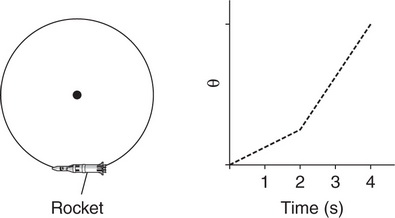# AP Physics 1 Question 161: Answer and Explanation

### Test Information

Question: 161

1.Question below refers to the following information: A bicycle wheel of known rotational inertia is mounted so that it rotates clockwise around a vertical axis, as shown in the first figure. Attached to the wheel’s edge is a rocket engine, which applies a clockwise torque τ on the wheel for a duration of 0.10 s as it burns. A plot of the angular position θ of the wheel as a function of time t is shown in the graph.

In addition to the wheel’s rotational inertia and the duration of time the engine burns, which of the following information from the graph would allow determination of the net torque the rocket exerts on the wheel?

• A. The area under the graph between t = 0 s and t = 3 s
• B. The change in the graph’s slope before and after t = 2 s
• C. The vertical axis reading of the graph at t = 3 s
• D. The vertical axis reading of the graph at t = 2 s